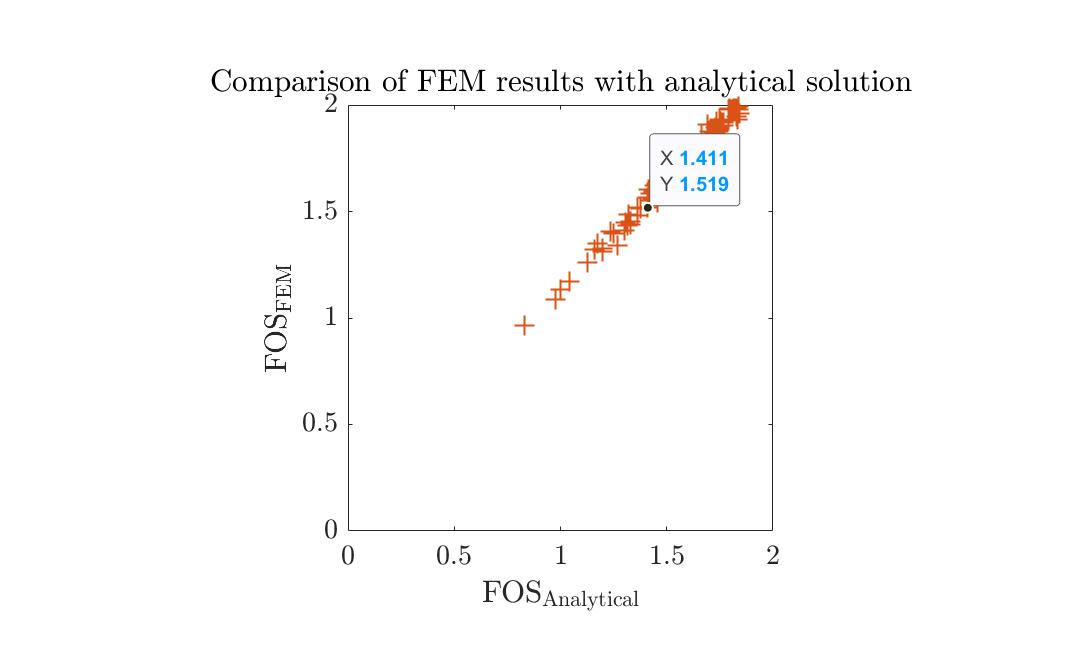# FORM analysis with FEM software and wrapper

Hello,

I’m performing a reliability analysis on the bearing capacity of a strip foundation. Failure is defined as the factor of safety FOS = Pcr/V being smaller than 2, so the limit state function becomes g = FOS - 2. Pcr is the critical load. It is calculated from a finite element software and depends on the soil cohesion c and friction angle \phi. V is the applied load but isn’t an input of my finite element software. My random input variables are so fi, c and V. The problem is, that the parser I use to extract the output of my finite element software returns Pcr and I have to calculate FOS in another step, taking also V into account. To solve this problem I defined a function FOS = STRIPmodel(X)(.m file, this function becomes my MODEL), which takes as input the vector X = [\phi, c, V], runs my FEM software thanks to UQLink, extract Pcr and at the end calculates FOS = Pcr/V. Everything works fine, the MCS and AKMCS probability of failure are 21.2% and 20.6%, respectively, but if I run FORM, I become a probability of failure of 1.4% and an importance factor of 1 for V. It is maybe dependent on the fact that I call my finite element software through a function? Below you can find some sections of my code.

% Forward model
mOpts.mFile = 'STRIPmodel';
mOpts.isVectorized = false;
myModel = uq_createModel(mOpts);

% Probabilistic input
IOpts.Marginals(1).Name = '$\phi$';
IOpts.Marginals(1).Type = 'LogNormal';
IOpts.Marginals(1).Moments = [30 0.08*30];
IOpts.Marginals(2).Name = 'c';
IOpts.Marginals(2).Type = 'LogNormal';
IOpts.Marginals(2).Moments = [5 0.2*5];
IOpts.Marginals(3).Name = 'V';
IOpts.Marginals(3).Type = 'Gumbel';
IOpts.Marginals(3).Moments = [1200 0.1*1200];
myInput = uq_createInput(IOpts);

% Reliability
FORMOptions.Type = 'Reliability';
FORMOptions.Method = 'FORM';
FORMOptions.Input = myInput;
FORMOptions.Model = myModel;
FORMOptions.LimitState.Threshold = 2;
FORMOptions.LimitState.CompOp = '<';
FORMAnalysis = uq_createAnalysis(FORMOptions);

The function (actually my MODEL), which runs my FEM software is defined as follows:

function FOS = STRIPmodel(X)

% UQLink ...

ZS.Output.Parser = 'readPcr_STRIP';
ZS.Output.FileName = {"File_to_read.his"};

% Create the UQLink wrapper
myUQLinkModel = uq_createModel(ZS);
Pcr = uq_evalModel(myUQLinkModel,X(:,1:2));

FOS = Pcr/X(3);

end

Thank you!

1 Like

Hi @Gil,

I think you set up your problem exactly as it should be.

I don’t see any reason this set-up would not work with FORM while working with MCS and AK-MCS. Do you have any warning while running FORM? More importantly, have you considered the possibility that FORM is not appropriate for solving this specific reliability problem? This may happen if your limit-state function is highly non-linear and/or if there exists multiple most probable failure points.

Cheers,
Moustapha

Dear @moustapha,

No, there aren’t warnings. If I run the same analysis, but as forward model I use the analytical solution instead of my FEM software, the FORM analysis works. Does maybe this behaviour mean, that the output of my FEM software depends “less linearly” on my input than the analytical solution? I tried to compare the analytical solution with the FEM results for a set of random variables and to me it seems there is good agreement between the two approaches. Below a figure of the comparison.